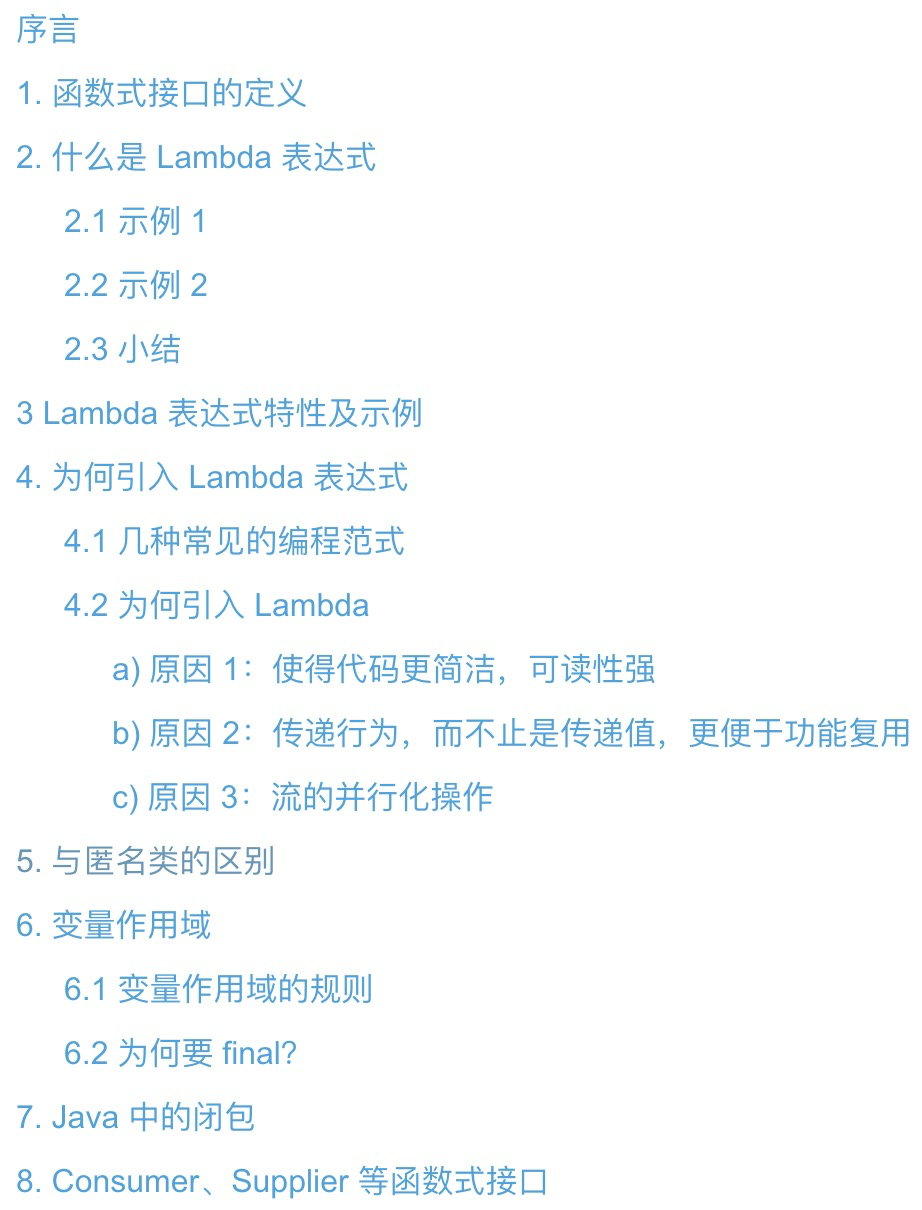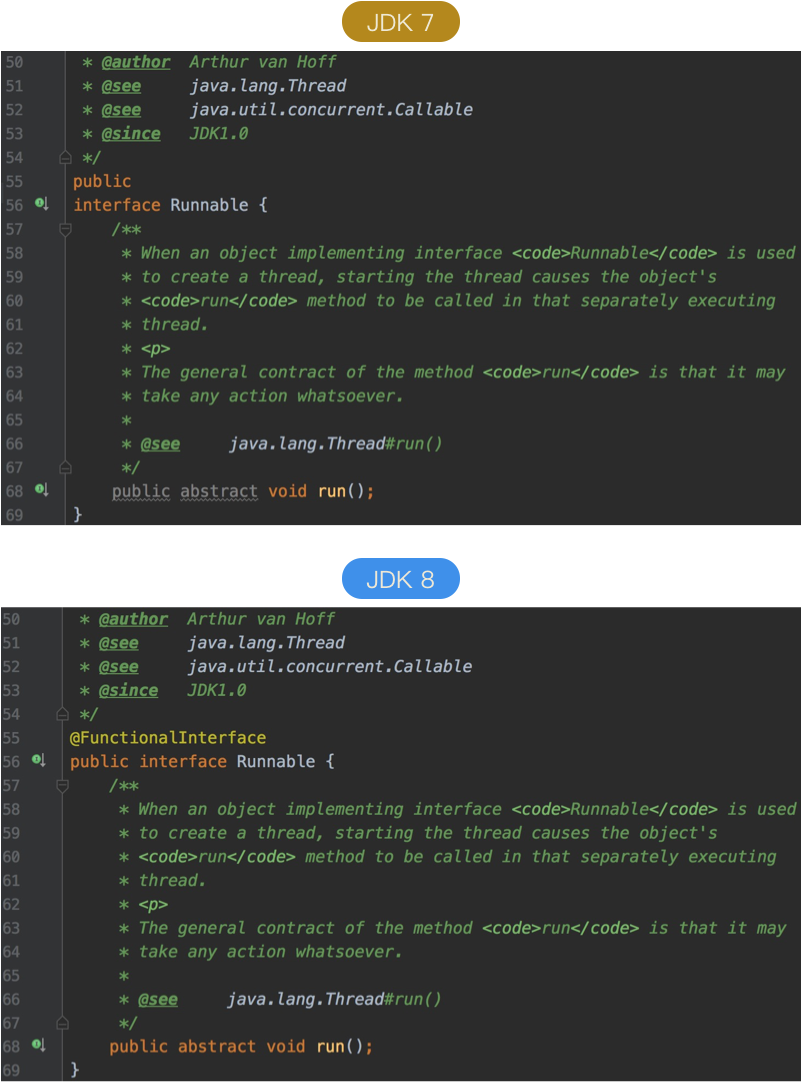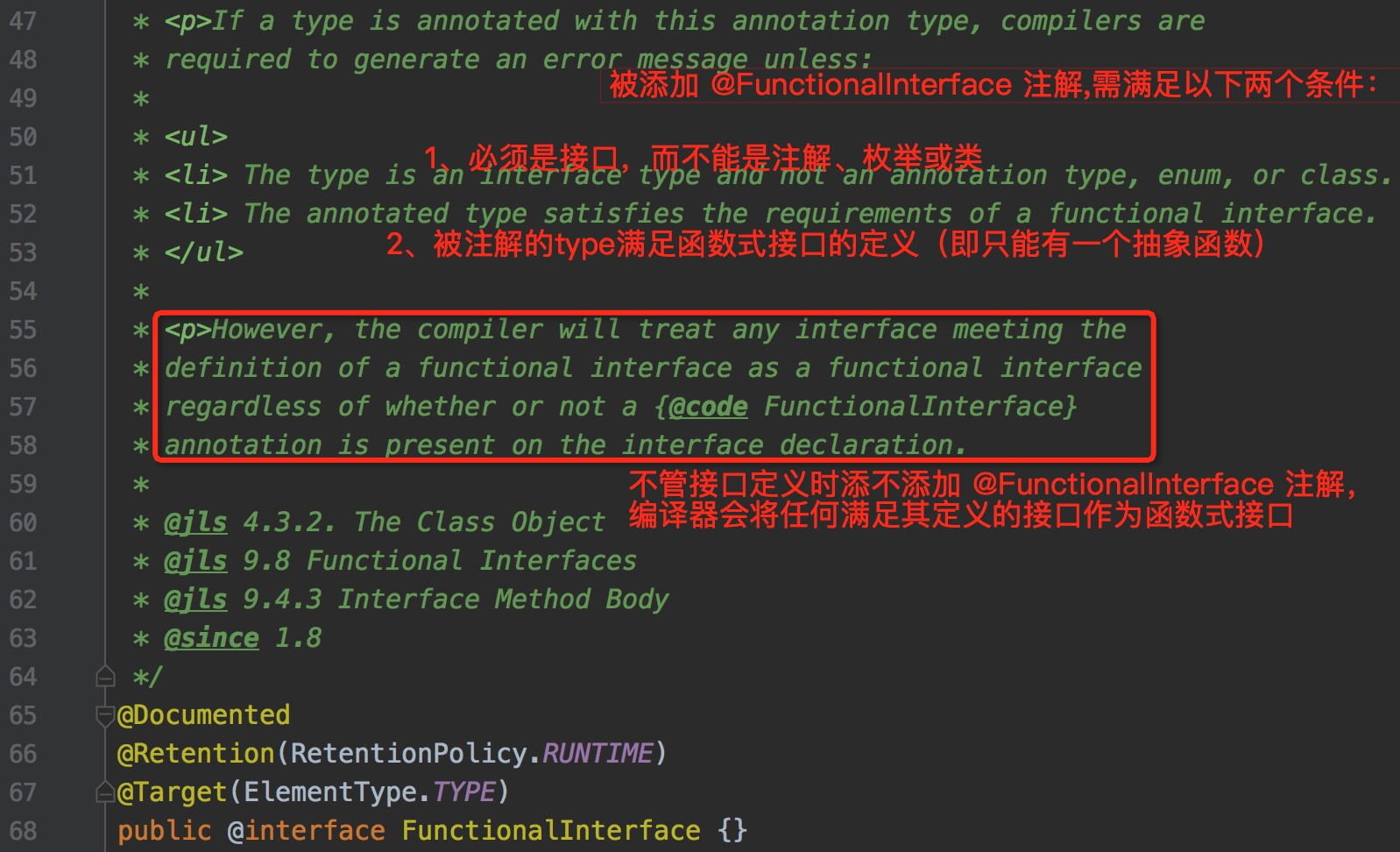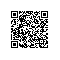# 深入探讨 Lambda 表达式（上）# 序言

JDK8 日渐成为项目开发中的主流。

## 1. 函数式接口的定义

a) 初级版：新增一个类，实现 Runnable 接口

/**
* @author: sss
*/
@Override
public void run() {
...
}
}

public class Main {
public static void main(String[] args) {
// new 一个新线程，执行任务A

// 主线程继续做其他事情
System.out.println("do other things...");
}
}


b) 进阶版：使用匿名内部类

public class Main {
public static void main(String[] args) {
// 通过匿名类来创建一个新线程，执行任务A
Runnable taskA = new Runnable() {
@Override
public void run() {
...
}
};

// 主线程继续做其他事情
System.out.println("do other things...");
}
}


c) 高级版：使用 Lambda 表达式

public static void main(String[] args) {
// 通过匿名类来创建一个新线程，执行任务A
// do things
...
}).start();

// 主线程继续做其他事情
System.out.println("do other things...");
}// 错误示例 1
@FunctionalInterface
interface InvalidInterfaceA {
}

// 错误示例 2
@FunctionalInterface
interface InvalidInterfaceB {
void testA();
void testB();
}


@FunctionalInterface
interface InvalidInterfaceC {
void testC();
}

@FunctionalInterface
interface InvalidInterfaceD {
void testD();
default void testE() {
System.out.println("this is a default method.");
}
}


@FunctionalInterface修饰的接口，只能有一个抽象方法，但并代表只能有一个方法声明，像上面的  InvalidInterfaceD 接口，还有 default 关键字修饰的 testE() 方法，但这是一个有默认实现的方法，并不是抽象方法，因此接口  InvalidInterfaceD  依然符合函数式接口的定义。• 必须是接口，不能是注解、枚举或类，限定了使用的类型范围
• 被注解的接口，必须满足函数式接口的定义，即只能有一个抽象函数

interface MathOperation {
int operation(int a, int b);
}

public static void main(String args[]) {
MathOperation addition = (int a, int b) -> a + b;
}


## 2. 什么是 Lambda 表达式

### 2.1 示例 1

public static void main(String[] args) {
// 使用匿名内部类
@Override
public void run() {
}
}).start();

// 使用 Lambda 表达式
}


### 2.2 示例 2

public static void main(String[] args) {
List<String> strList = Arrays.asList("a", "b", "c", "d");
// 方式1
for (int i = 0; i < strList.size(); i++) {
System.out.println(strList.get(i));
}
// 方式2，语法糖，本质还是下面的方式3
for (String str : strList) {
System.out.println(str);
}
// 方法3
Iterator<String> iterator = strList.iterator();
while (iterator.hasNext()) {
System.out.println(iterator.next());
}
}


strList.forEach(str -> System.out.println(str));


default void forEach(Consumer<? super T> action) {
Objects.requireNonNull(action);
for (T t : this) {
action.accept(t);
}
}

/**
* Represents an operation that accepts a single input argument and returns no
* result. Unlike most other functional interfaces, {@code Consumer} is expected
* to operate via side-effects.
*
* <p>This is a <a href="package-summary.html">functional interface</a>
* whose functional method is {@link #accept(Object)}.
*
* @param <T> the type of the input to the operation
*
* @since 1.8
*/
@FunctionalInterface
public interface Consumer<T> {
void accept(T t);

default Consumer<T> andThen(Consumer<? super T> after) {
Objects.requireNonNull(after);
return (T t) -> { accept(t); after.accept(t); };
}
}


forEach()  的形参是一个 Consumer 对象，而  Comsumer  接口又是一个有 @FunctionalInterface 注解的函数式接口，其抽象方法是  accept(T t)

strList.forEach(new Consumer<String>() {
@Override
public void accept(String str) {
System.out.println(str);
}
});


strList.forEach(str -> System.out.println(str));


### 2.3 小结

() -> {...}

(xxx) -> {...}


## 3 Lambda 表达式特性及示例

• 特性 1：由箭头将表达式分为左、右两个部分

• 特性 2：入参可为零个、一个、多个

() -> {System.out.println("test expression!");};
() -> 123;


(x) -> {System.out.println(x);};
x => x + 2;


(x, y, z) -> {
System.out.println(x);
System.out.println(y);
System.out.println(z);
};


• 特性 3：入参类型声明可省略，编译器会做自动类型推断
List<String> strList = Arrays.asList("a", "b", "c", "d");
strList.forEach(str -> {
System.out.println(str);
});


• 特性 4：表达式右侧的 body 中，只有一条语句，则可省略大括号，否则不可省略

strList.forEach(str -> System.out.println(str));

• 特性 5：表达式的返回值是可选的

## 4.  为何引入 Lambda 表达式

### 4.1 几种常见的编程范式

## 找出所有学生的数学成绩
select name,
age,
course,
score
from student
where course= "math";


def sum(x):
return x + y;

sum2 = sum(2);
elementB = sum(7);
a = sum2(3); # 2 + 3 = 5
b = elementB(1); # 7 + 1 = 8
print a; # 输出5
print b; # 输出8


public int sum(int x, int y) {
return x + y;
}


### 4.2 为何引入 Lambda

#### a) 原因 1：使得代码更简洁，可读性强

new Thread(() -> System.out.println("this is a Lambda expression!")).start();


Thread 类的有参构造函数 Thread(Runnable runnable)，本来参数是一个 Runnable 对象，

List<String> nameList = Arrays.asList("tom", "kate", "jim", "david");
List<String> newNameList = nameList
.stream()
.filter(name -> name.length() > 3)
.map(name -> name.toUpperCase())
.sorted()
.collect(Collectors.toList());


#### b) 原因 2：传递行为，而不止是传递值，更便于功能复用

// 给定一个整数集合
List<Integer> list = Arrays.asList(1, 2, 3, 4, 5, 6, 7, 8, 9);

// 求所有元素的和
private Integer sumAll(List<Integer> list) {
int sum = 0;
for (Integer ele : list) {
sum += ele;
}
return sum;
}

// 求所有偶数元素的和
private Integer sumEven(List<Integer> list) {
int sum = 0;
for (Integer ele : list) {
if (ele % 2 == 0) {
sum += ele;
}
}
return sum;
}

// 求所有奇数元素的和
private Integer sumOdd(List<Integer> list) {
int sum = 0;
for (Integer ele : list) {
if (ele % 2 == 1) {
sum += ele;
}
}
return sum;
}
// 求所有大于3的元素的和
private Integer sumLargerThan3(List<Integer> list) {
int sum = 0;
for (Integer ele : list) {
if (ele > 3) {
sum += ele;
}
}
return sum;
}


public interface sumStrategy {
Integer sum(List<Integer> list);
}

public class SumAllStrategy implements sumStrategy {
@Override
public Integer sum(List<Integer> list) {
int sum;
for (Integer ele : list) {
sum += ele;
}
return sum;
}
}

public class SumEvenStrategy implements sumStrategy {
@Override
public Integer sum(List<Integer> list) {
...
}
}

// 实际调用
List<Integer> list = Arrays.asList(1, 2, 3, 4, 5, 6, 7, 8, 9);
// 示例1：当想求所有元素的和时，使用 SumAllStrategy 类
Strategy strategy1 = new SumAllStrategy();
strategy1.sum(list);

// 示例2：当想求所有偶数元素的和时，使用 SumEvenStrategy 类
Strategy strategy2 = new SumEvenStrategy();
strategy2.sum(list);


// @since 1.8
@FunctionalInterface
public interface Predicate<T> {
/**
* Evaluates this predicate on the given argument.
*
* @param t the input argument
* @return {@code true} if the input argument matches the predicate,
* otherwise {@code false}
*/
boolean test(T t);

...
}


Lambda 的使用如下：

private Integer sum(List<Integer> list, Predicate condition) {
int sum = 0;
for (Integer ele : list) {
if (condition.test(ele)) {
sum += ele;
}
}
return sum;
}

// 实际使用
List<Integer> list = Arrays.asList(1, 2, 3, 4, 5, 6, 7, 8, 9);
// 示例1：求所有元素的和
int sum = sum(list, x -> true);
// 示例2：求所有偶数元素的和
sum = tester.sum(list, x -> (int)x % 2 == 0);
// 示例3：求所有大于5的元素的和
sum = tester.sum(list, x -> (int)x > 5);


#### c) 原因 3：流的并行化操作

List<Integer> list = Arrays.asList(1, 2, 3, 4, 5, 6, 7, 8, 9);
// 串行
Integer reduce = list.stream().reduce((a, b) -> a + b).get();
// 并行，相比串行多了 parallel() 函数
Integer reduce = list.stream().parallel().reduce((a, b) -> a + b).get();


## 小结

• Lambda 表达式和匿名内部类的区别？
• 变量作用域
• Java 中的闭包是什么？
• 常用的 Consumer、Supplier 等函数式接口怎么用？